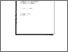# Symmetry and Extensions of Arrow's Theorem

Saari, D.G. (1987). Symmetry and Extensions of Arrow's Theorem. IIASA Working Paper. IIASA, Laxenburg, Austria: WP-87-109Preview
Text
WP-87-109.pdf

## Abstract

By emphasizing the symmetry of certain set theoretic conditions, shown to be associated with Arrow's Impossibility Theorem, a characterization of "kinds of axioms" is obtained. More precisely, if the defining properties of a model satisfies these conditions, then the model must have a conclusion much like that of Arrow's theorem. Because the conditions are described in set theoretic terms, the applicability of these results extends beyond the usual setting of complete, binary, transitive rankings to space of utility functions, probability distributions, etc. In this manner, not only can new extensions of Arrow's theorem be obtained, but it is shown how the same "kinds of axioms" applies to, say, problems about the aggregate excess demand function, the Hurwicz-Schmeidler dictatorial result about Pareto optimal, Nash equilibria, the Gibbard-Satterthwaite theorem about manipulability, etc.

Item Type: Monograph (IIASA Working Paper) System and Decision Sciences - Core (SDS) IIASA Import 15 Jan 2016 01:57 27 Aug 2021 17:12 http://pure.iiasa.ac.at/2943View Item